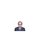# Tackling Algebra

I AM UNABLE TO TACKLE THIS PROBLEM. CAN ANYONE COME FORWARD TO HELP ME.

a+b+c=-1/2,ab+bc+ca=-1/2,abc=1/8, then how to get 1) 1/a+1/b+1/c and 2)a^1/3+b^1/3+c^1/3.Note by Prabir Chaudhuri
6 years, 6 months ago

This discussion board is a place to discuss our Daily Challenges and the math and science related to those challenges. Explanations are more than just a solution — they should explain the steps and thinking strategies that you used to obtain the solution. Comments should further the discussion of math and science.

When posting on Brilliant:

• Use the emojis to react to an explanation, whether you're congratulating a job well done , or just really confused .
• Ask specific questions about the challenge or the steps in somebody's explanation. Well-posed questions can add a lot to the discussion, but posting "I don't understand!" doesn't help anyone.
• Try to contribute something new to the discussion, whether it is an extension, generalization or other idea related to the challenge.

MarkdownAppears as
*italics* or _italics_ italics
**bold** or __bold__ bold
- bulleted- list
• bulleted
• list
1. numbered2. list
1. numbered
2. list
Note: you must add a full line of space before and after lists for them to show up correctly
paragraph 1paragraph 2

paragraph 1

paragraph 2

[example link](https://brilliant.org)example link
> This is a quote
This is a quote
    # I indented these lines
# 4 spaces, and now they show
# up as a code block.

print "hello world"
# I indented these lines
# 4 spaces, and now they show
# up as a code block.

print "hello world"
MathAppears as
Remember to wrap math in $$ ... $$ or $ ... $ to ensure proper formatting.
2 \times 3 $2 \times 3$
2^{34} $2^{34}$
a_{i-1} $a_{i-1}$
\frac{2}{3} $\frac{2}{3}$
\sqrt{2} $\sqrt{2}$
\sum_{i=1}^3 $\sum_{i=1}^3$
\sin \theta $\sin \theta$
\boxed{123} $\boxed{123}$

Sort by:

1/a+1/b+1/c=(ab+bc+ca)/abc

- 5 years, 9 months ago

Let $a^{\frac{1}{3}} = x, b^{\frac{1}{3}} = y ,c^{\frac{1}{3}} = z$

then we have

$\begin{cases} x^3+y^3+z^3=-\dfrac{1}{2}\\ (xy)^3+(yz)^3+(xz)^3=-\dfrac{1}{2}\\ (xyz)^3=\dfrac{1}{8} \end{cases}$

using the identity

$A^3+B^3+C^3=(A+B+C)^3-3(A+B+C)(AB+BC+AC)+3ABC$

we have,

$\begin{cases} (x+y+z)^3-3(x+y+z)(xy+yz+xz)+3xyz=-\dfrac{1}{2}\\ (xy+yz+xz)^3-3(xy+yz+xz)[xyz(x+y+z)]+3x^2y^2z^2=-\dfrac{1}{2}\\ xyz=\dfrac{1}{2} \end{cases}$

Now,

Let $u=x+y+z, v=xy+yz+xz$

then we have

$\begin{cases} u^3-3uv+2=0\\ 4v^3-6uv+5=0 \end{cases}$

$\Longrightarrow 4v^3-2u^3+1=0, v=\dfrac{u^3+2}{3u}$

so

$4\left(\dfrac{u^3+2}{3u}\right)^3-2u^3+1=0\Longrightarrow 4u^9-30u^6+75u^3+32=0$

let $t=u^3$, so we have

$4t^3-30t^2+75t+32=0$

let $t=\dfrac{5}{2}-a$,then

$4\left(\dfrac{5}{2}-a\right)^3-30\left(\dfrac{5}{2}-a\right)^2+75\left(\dfrac{5}{2}-a\right)+32=0$

$\Longrightarrow 4a^3=\dfrac{189}{2}\Longrightarrow a=\dfrac{3\sqrt{7}}{2}$

$\Longrightarrow t=\dfrac{5}{2}-\dfrac{3\sqrt{7}}{2}$

Therefore,

$a^{\frac{1}{3}} + b^{\frac{1}{3}} + c^{\frac{1}{3}} = x+y+z = u = t^{\frac{1}{3}} = \sqrt{\dfrac{1}{2}\left(5-3\sqrt{7}\right)}$

- 6 years, 5 months ago

@PRABIR CHAUDHURI I have posted my solution of the other part. You may look at it.

- 6 years, 5 months ago

See.. the first part is easy as ab+bc+ca=-1/2divide the equation by abc which is also equal to 1/8 . We get 1/a +1/b +1/c=1/2 * 8 =4.

- 6 years, 6 months ago

Thanks.

- 6 years, 6 months ago